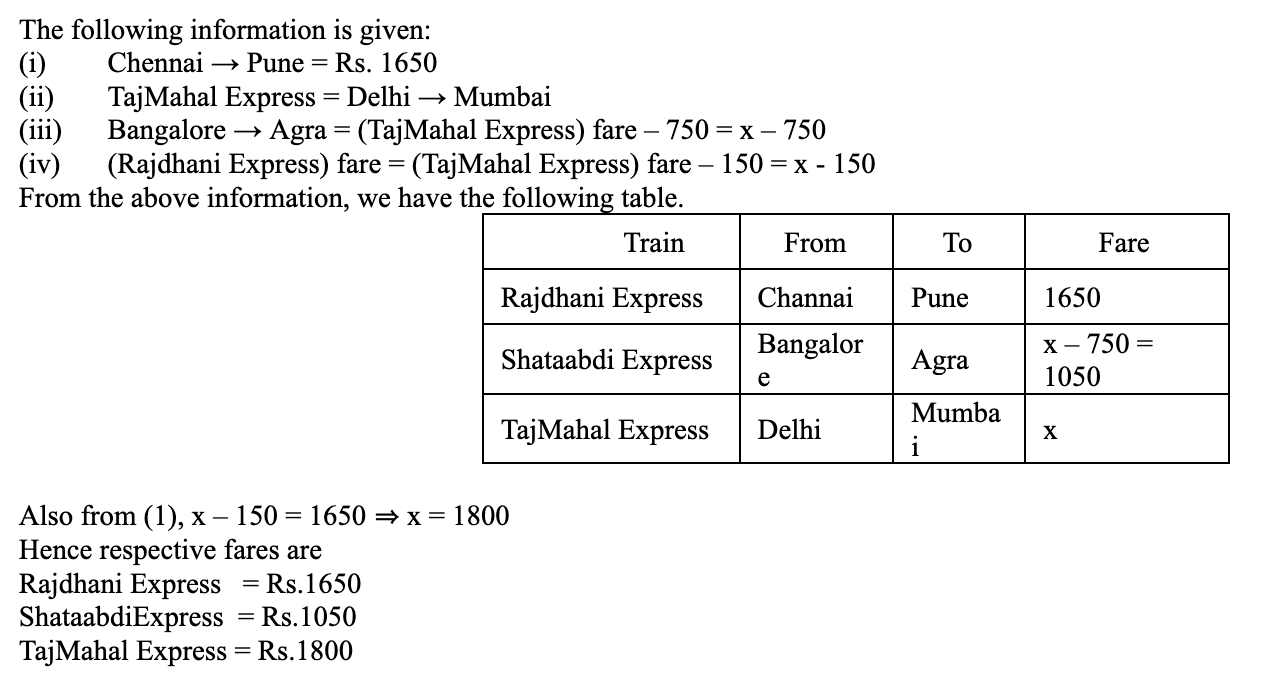CAT | TCS Logical Questions

## TCS Logical Questions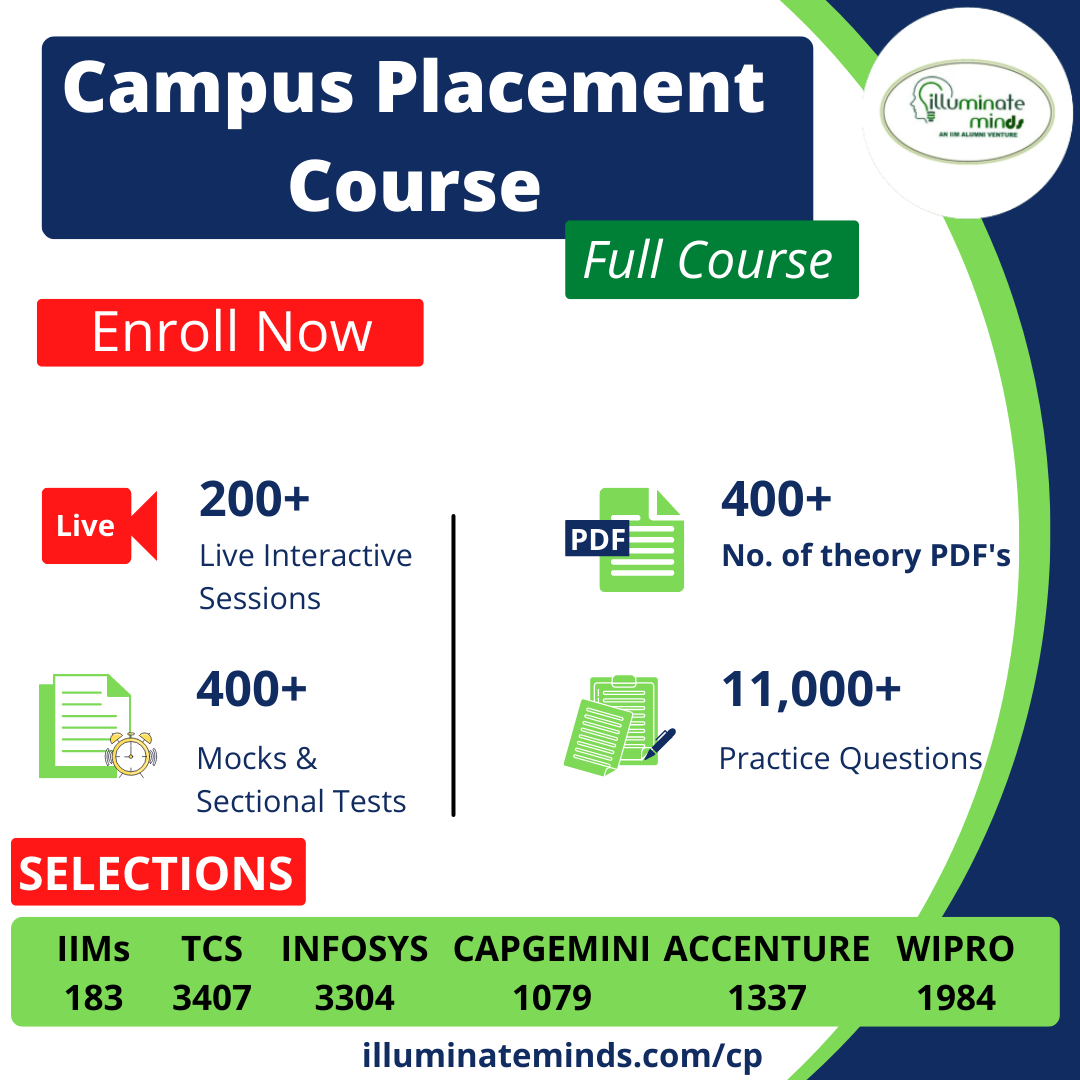Jobs By Batch
Jobs By Location
Jobs By Degree
Jobs By Branch
IT Jobs
Internships
Govt. Jobs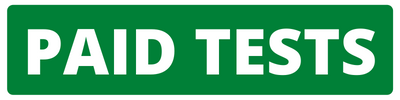• ##### Original Questions of IT Companies| All Topics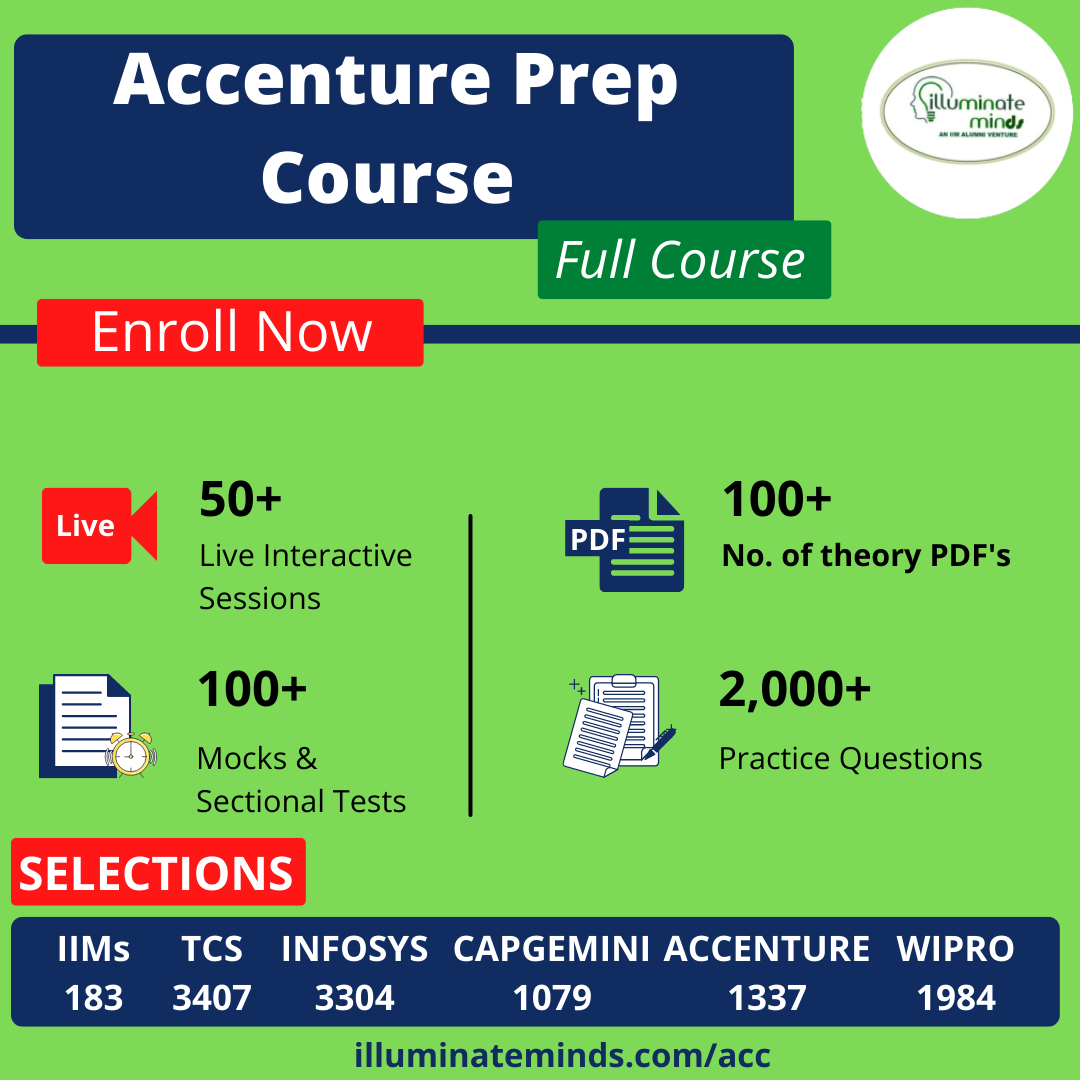### Quiz Begins Here

Q #1
:

#### Logical Question

Directions: These questions are based on the following pie chart and the table, which gives the details of expenses of an Excellent Management Training Institute in India (EMTI)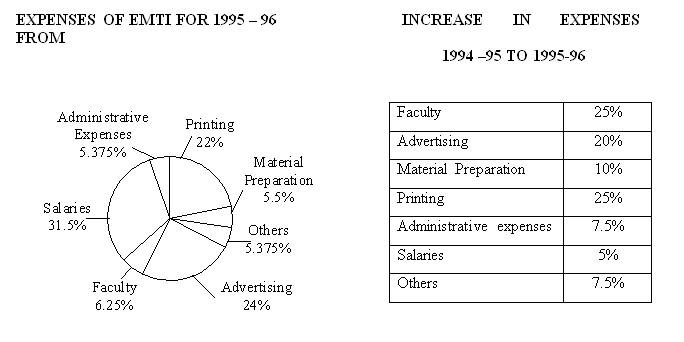If the profits were 20% of total turnover in 1995-96, and total expenses in 1995-96 are the same as the turnover of 1994-95, profit as a percentage of turnover in 1994-95 is how many percentage points less than that in 1995-96?
+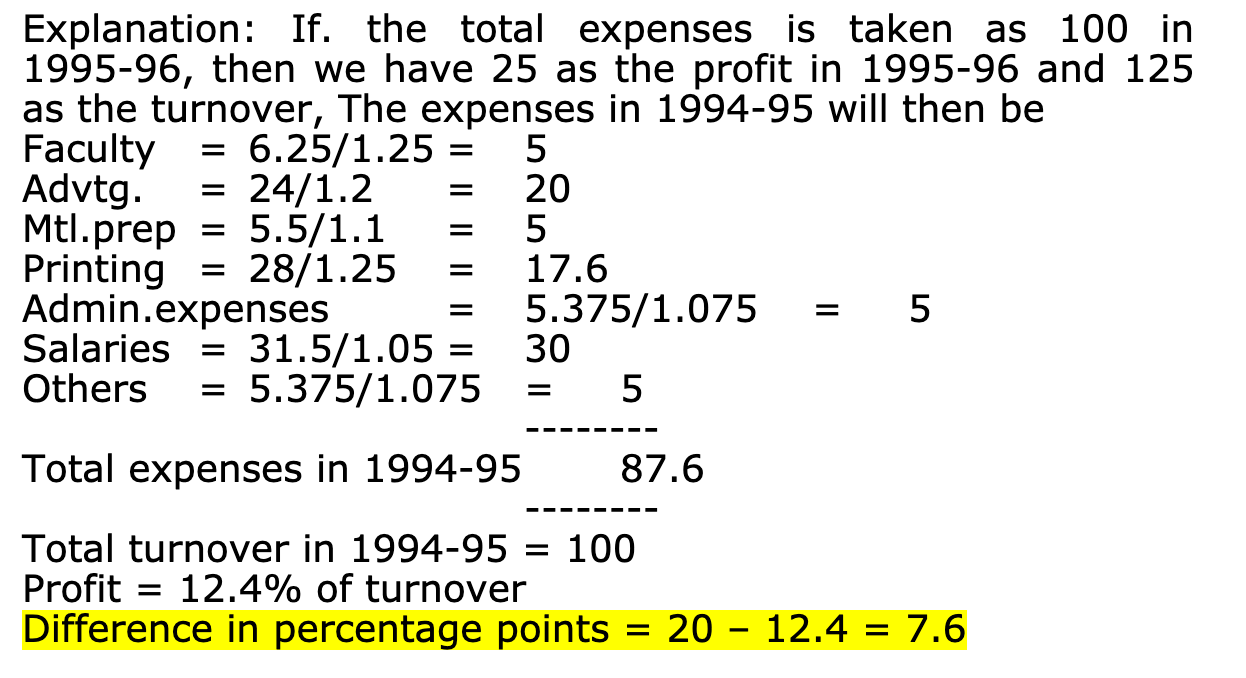Q #2
:

#### Logical Question

Directions: These questions are based on the pie charts given below.The number of Color Televisions sold in India was 35 lakhs in the year 1999. The CTV market has been growing at a rate of 30% p.a every year and the trend has continued since 1999.

If ‘Others’ have a share of 25% in 2001 and the normal growth rate prevails in the market, what is the average number of CTVs sold in a month by these ‘Other’ companies (approximately)?
+

Explanation: 2 Total sales by others in 2001 = 35 x 1.30 x 1.30 x 105 x 0.25 ≅1.48 x 106 ∴average monthly sales = 123229

Q #3
:

#### Logical Question

Directions: These questions are based on the following tables which show the statistics of �Madras Textile Co.�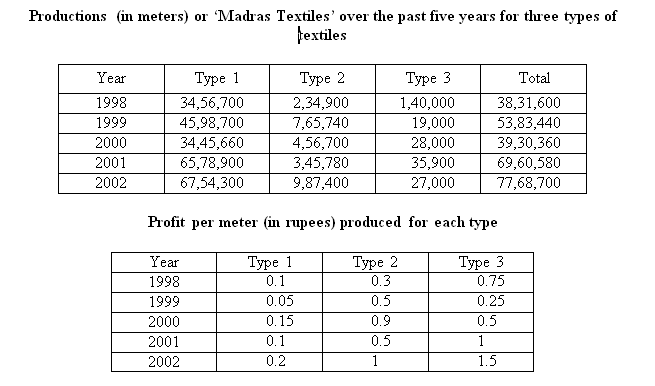What is the percentage increase in the total profit from the year 2001 to the year 2002?
+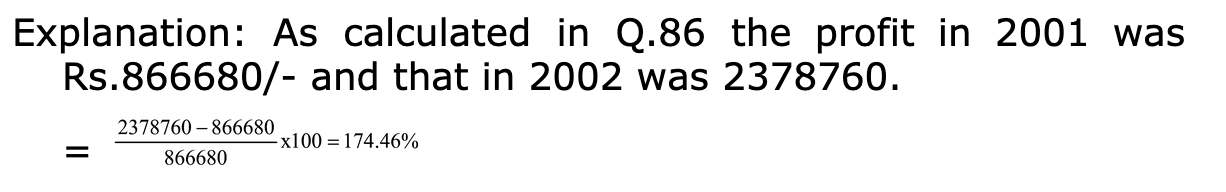Q #4
:

#### Logical Question

Directions: Refer the following data to answer the questions given below.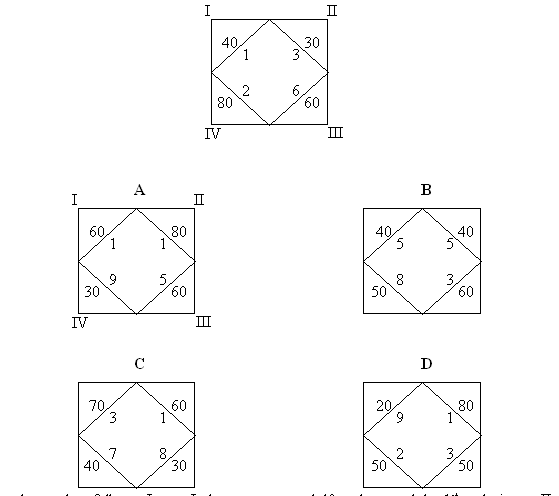The following grid show the marks scored with ranks of a student in 4 tests I, II, III, and IV. Initially the marks were entered in a grid such as the one shown below with marks and rank of subject 1 on the top left, II on the top right, etc.�

The data is to be read as follows. In test I, the person scored 40 and secured the 1st rank, in test II the person scored 30 and secured 3rd rank, etc.

Using a concept similar to the one above, marks of 4 students A, B, C and D were entered in 4 grids as shown below. However, in the following grids, the subjects for B, C and D have been erased and these 3 grids have been turned clockwise by 90�, 180� and 270�, not necessarily in that order. There are 10 students in the class, and in no subjects do any two students score the same marks. The more marks a person scores, the higher his rank in the subject. A scored the first rank in 2 subjects, while C and D scored the first rank in 1 subject each.

What was the total marks received by the four students together in subjects II?
+

Let us assume that grid of A is fixed. So in the subject that A scores 60 and secures rank 1, others must have scored less than 60. For B it could be marks 40, 40 or 50, for C it could be 40 or 30, and for D the corresponding subject mark could be 20, 50 ro 50. Similarly, in the subject that C scored the 1st rank, it is clear that A should have scored less than 60 i.e. A has scored 90 in that subject. So the mark of A aligned to mark of C would be as follows. Now, we know that in the subject that a scores 60 and secures 5th rank, C is not the 1st rank. Hence D must be the 1st rank in that subject. We can now align all subjects of all students accordingly.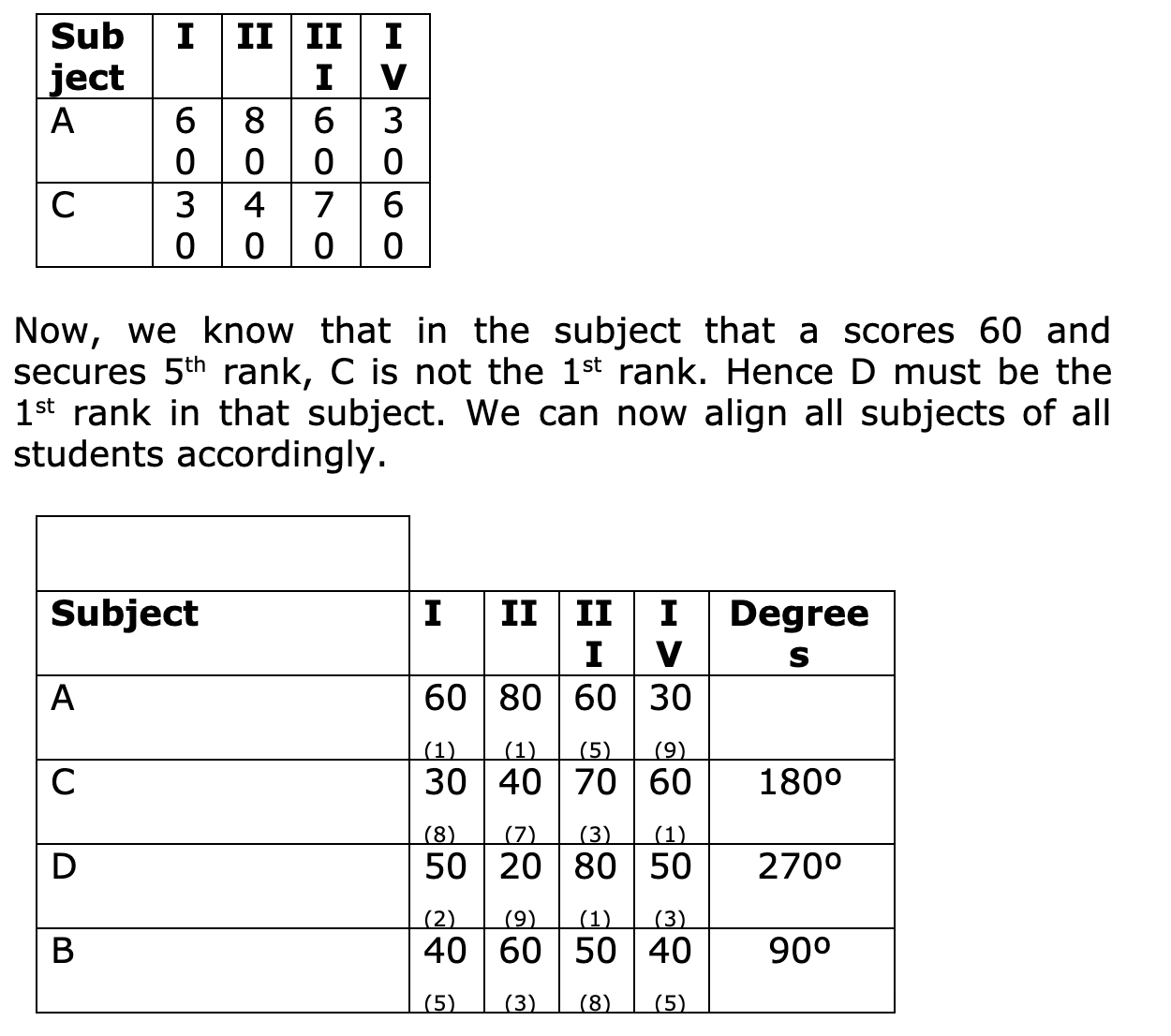Q #5
:

#### Logical Question

<b>Directions:</b> Three trains – Rajdhani Express, Shataabdi Express and TajMahal Express – travel between two stations without stopping anywhere in between. No two trains have the same starting station or the same terminating station or the same travel fare. Also, the following is known about these trains.

1. The fare for the train which travels between. Chennai and Pune is Rs. 1650.
2. TajMahal Express runs between Delhi and Mumbai.
3. Fare for the train which travels between Bangalore and Agra is Rs.750 less than the fare for TajMahal Express.
4. The fare for Rajdhani Express is Rs.150 less than the fare for TajMahal Express.

Which among the following statements is definitely true?
+